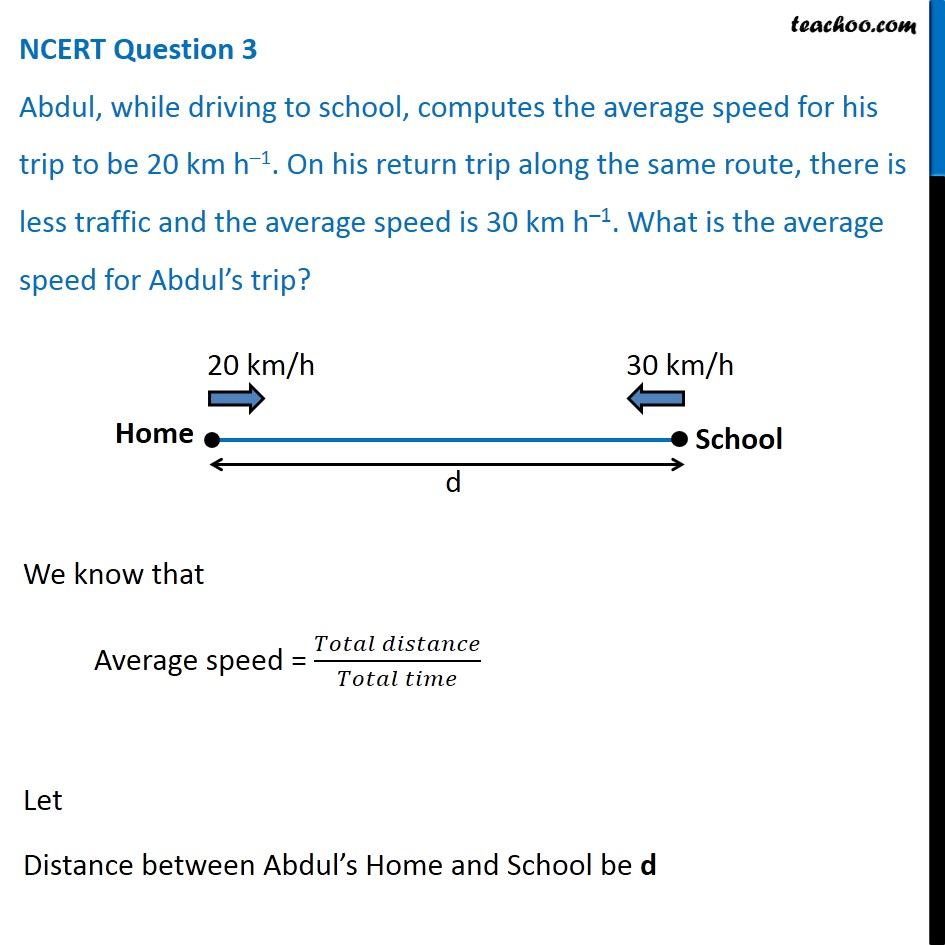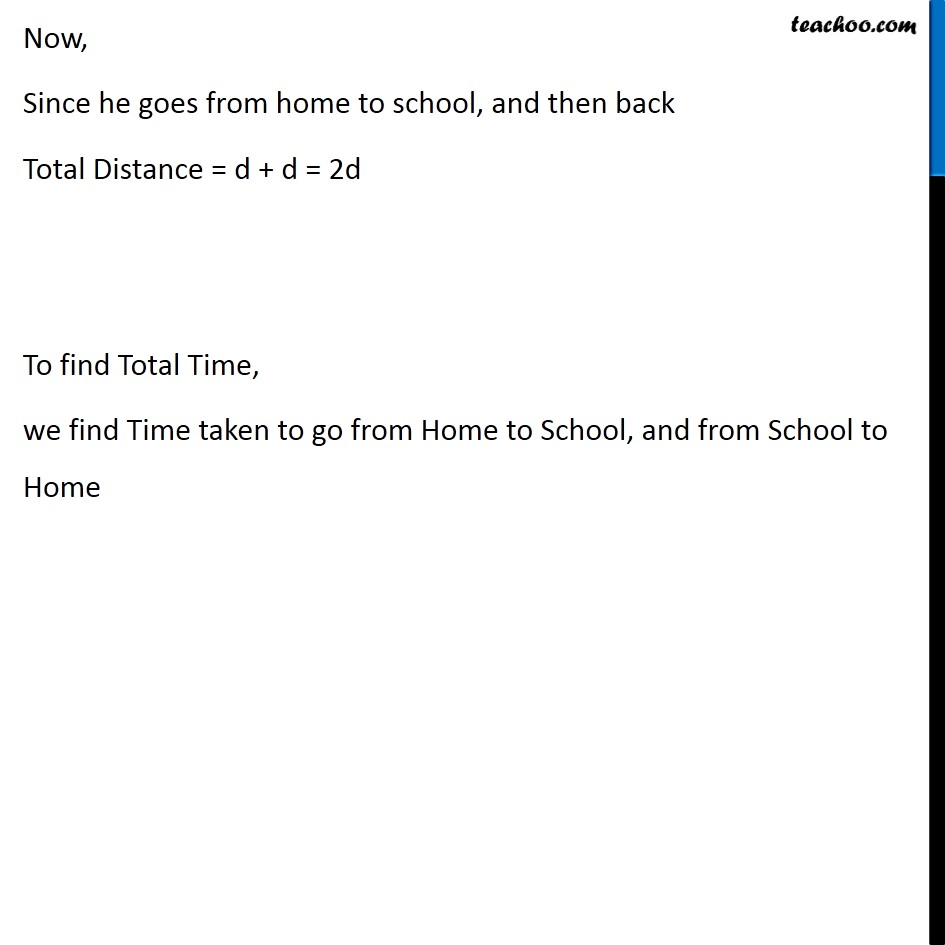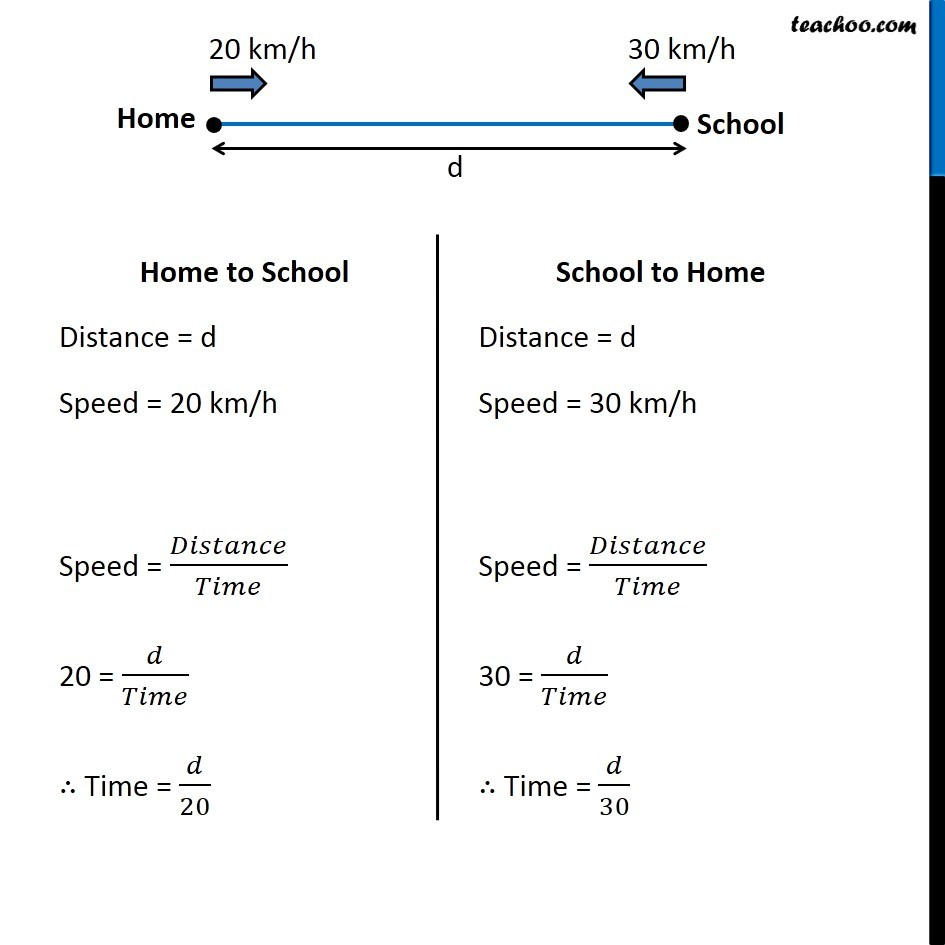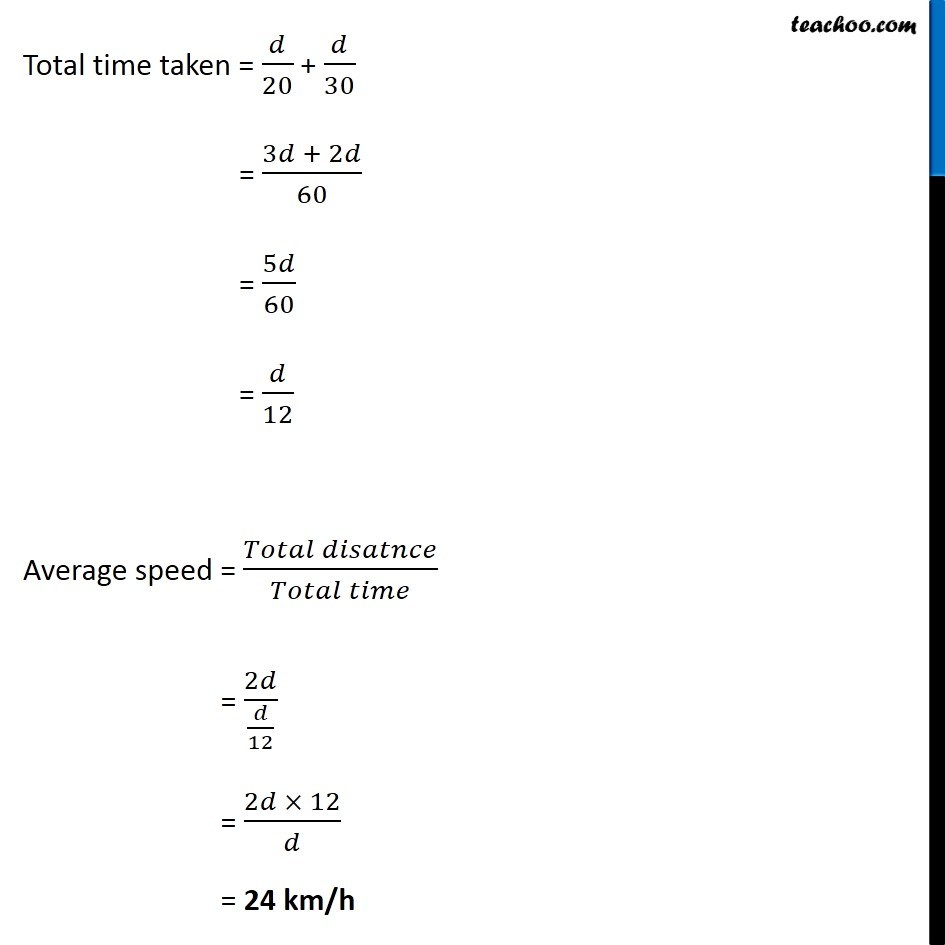NCERT Questions

Class 9
Chapter 8 Class 9 - MotionLearn in your speed, with individual attention - Teachoo Maths 1-on-1 Class

### Transcript

NCERT Question 3 Abdul, while driving to school, computes the average speed for his trip to be 20 km h–1. On his return trip along the same route, there is less traffic and the average speed is 30 km h–1. What is the average speed for Abdul’s trip? We know that Average speed = (𝑇𝑜𝑡𝑎𝑙 𝑑𝑖𝑠𝑡𝑎𝑛𝑐𝑒)/(𝑇𝑜𝑡𝑎𝑙 𝑡𝑖𝑚𝑒) Let Distance between Abdul’s Home and School be d Now, Since he goes from home to school, and then back Total Distance = d + d = 2d To find Total Time, we find Time taken to go from Home to School, and from School to Home Let time taken to reach home = 𝑡_2 Home to School Distance = d Speed = 20 km/h Speed = 𝐷𝑖𝑠𝑡𝑎𝑛𝑐𝑒/𝑇𝑖𝑚𝑒 20 = 𝑑/𝑇𝑖𝑚𝑒 ∴ Time = 𝑑/20 School to Home Distance = d Speed = 30 km/h Speed = 𝐷𝑖𝑠𝑡𝑎𝑛𝑐𝑒/𝑇𝑖𝑚𝑒 30 = 𝑑/𝑇𝑖𝑚𝑒 ∴ Time = 𝑑/30 Total time taken = 𝑑/20 + 𝑑/30 = (3𝑑 + 2𝑑)/60 = 5𝑑/60 = 𝑑/12 Average speed = (𝑇𝑜𝑡𝑎𝑙 𝑑𝑖𝑠𝑎𝑡𝑛𝑐𝑒)/(𝑇𝑜𝑡𝑎𝑙 𝑡𝑖𝑚𝑒) = 2𝑑/(𝑑/12) = (2𝑑 × 12)/𝑑 = 24 km/h